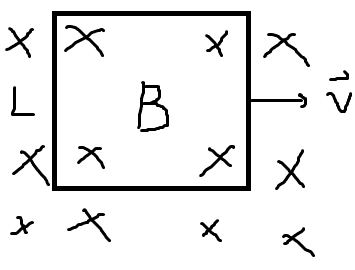# Work done on a moving square wire loop in a B-field

feynman1There’s a current i in the loop in the figure. The Ampere’s force iLB on a wire of length L exerts on charges in the wire but it does no work on the charges. The charges would go in circular motion if there were no wire. Then the wire exerts exactly iLB on those charges to keep the charges moving at constant speed. Then likewise the charges exert iLB on the wire. Then it is the charges that are doing work iLBv on the wire?

#### Attachments

Last edited by a moderator:

Mentor
As the loop moves to the right on both the right and left sides there is a large ##\partial \vec B/\partial t## and therefore by Faraday’s law we get a non zero ##\vec E## field.

feynman1
As the loop moves to the right on both the right and left sides there is a large ##\partial \vec B/\partial t## and therefore by Faraday’s law we get a non zero ##\vec E## field.
i changed that pic so that there's no change in B

Mentor
i changed that pic so that there's no change in B
You can change the pic, but now your pic doesn’t represent reality. If there is no change in the magnetic field then the wire cannot be carrying current.

•Gold Member
2022 Award
I don't know, whether I read your drawing correctly: If the entire loop moves in a homogeneous magnetic field there's indeed no work done. It just moves with constant velocity.

The usual setup is shown here in the figure with the resistor (the explanations on the webpage are not optimal, to say it friendly):

http://labman.phys.utk.edu/phys222core/modules/m5/motional_emf.html

Take the situation in this figure as the initial condition and let the wire just run without any external force. Then we can make a simple analysis to see how work is done here. We use the quasistationary approximation.

The motion of the wire induces an electromotive force according to Faraday's Law (in SI units)
$$\mathcal{E}=-\dot{\Phi}=v B d.$$
According to Ohm's law this induces a current going upwards in the wire
$$i=\mathcal{E}/R=v B d/R.$$
Now due to this current there acts a force to left on the wire, i.e.,
$$m \dot{v}=-B i d=-\frac{B^2 d^2}{R} v.$$
The power is
$$P=m \dot{v} v = -\frac{B^2 d^2}{R} v^2.$$
This is precisely the heat due to the ohmic losses in the wire,
$$P_\text{ohm}=R i^2=\frac{B^2 d^2}{R} v^2.$$
So the work done here is due to friction of the charges in the wire. It's not the magnetic field directly doing any work. It cannot do work, because the force on each charge is always perpendicular to its velocity.

Staff Emeritus
It's pretty obnoxious to ask a question, get an answer, and then change the question by editing it. That just confuses everybody.

As pointed out, there's no work done. If you draw the system at two different times, nothing changes: you could take two snapshots and overlay them so the loops are in the same place and there is otherwise no difference.

•weirdoguy
Staff Emeritus
i changed that pic so that there's no change in B

It's pretty obnoxious to ask a question, get an answer, and then change the question by editing it. That just confuses everybody.

@Vanadium 50 is right. @feynman1, the correct way to do it is to add a new post with the correction and not edit the original, especially after getting an answer.

feynman1
how to delete the original post

Staff Emeritus
how to delete the original post
You don't. I don't think you see the point here.

The original post #1 asks a question. An answer #2 is provided. If you then edit or delete #1, then #2 looks silly and hard to explain. It would be unfair to the author of #2.

•